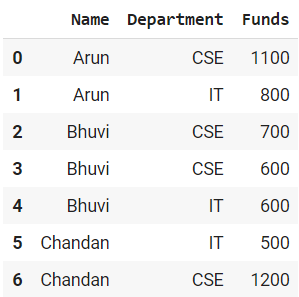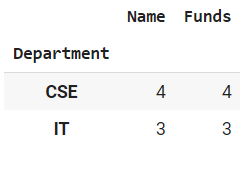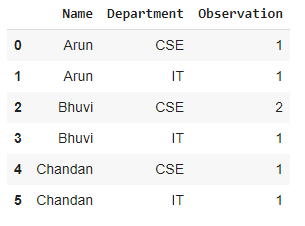GeeksforGeeks App
Open AppBrowser
Continue

# How to Count Observations by Group in Pandas?

In real data science projects, you’ll be dealing with large amounts of data and trying things over and over, so for efficiency, we use the Groupby concept. Groupby concept is really important because its ability to aggregate data efficiently, both in performance and the amount code is magnificent. Groupby mainly refers to a process involving one or more of the following steps they are:

• Splitting: It is a process in which we split data into groups by applying some conditions on datasets.
• Applying: It is a process in which we apply a function to each group independently.
• Combining: It is a process in which we combine different datasets after applying groupby and results into a data structure.

Syntax : groupby(by=None, axis=0, level=None, as_index=True, sort=True, group_keys=True, squeeze=False, **kwargs)

Parameters :

• by : mapping, function, str, or iterable
• axis : int, default 0
• level : If the axis is a MultiIndex (hierarchical), group by a particular level or levels
• as_index : For aggregated output, return object with group labels as the index. Only relevant for DataFrame input. as_index=False is effectively “SQL-style” grouped output
• sort : Sort group keys. Get better performance by turning this off. Note this does not influence the order of observations within each group. groupby preserves the order of rows within each group.
• group_keys : When calling apply, add group keys to index to identify pieces
• squeeze : Reduce the dimensionality of the return type if possible, otherwise return a consistent type

Returns : GroupBy object

Here, we use a simple dummy dataframe which is shown below:Also, we use some methods to count the observations by the group in Pandas which are explained below with examples.

Example 1: Using group.count (Count By One Variable)

In this example, we will use group.count() method which counts the total number of members in each group.

## Python3

 `# import libraries``import` `pandas as pd`` ` `#create pandas DataFrame``df ``=` `pd.DataFrame({``'Name'``: [``'Arun'``, ``'Arun'``, ``'Bhuvi'``, ``'Bhuvi'``,``                            ``'Bhuvi'``, ``'Chandan'``, ``'Chandan'``],``                    ` `                   ``'Department'``:[``'CSE'``, ``'IT'``, ``'CSE'``, ``'CSE'``,``                                 ``'IT'``, ``'IT'``, ``'CSE'``],``                    ` `                   ``'Funds'``: [``1100``, ``800``, ``700``, ``600``, ``600``, ``500``, ``1200``]})`` ` `# create a group using groupby``group ``=` `df.groupby(``"Department"``)`` ` `# count the observations``group.count()`

Output:Example 2: Using group.size (Count By Multiple Variables)

In this example, we will use group.size() method which counts the number of entries/rows in each group.

## Python3

 `# import libraries``import` `pandas as pd`` ` `#create pandas DataFrame``df ``=` `pd.DataFrame({``'Name'``: [``'Arun'``, ``'Arun'``, ``'Bhuvi'``, ``'Bhuvi'``, ``                            ``'Bhuvi'``, ``'Chandan'``, ``'Chandan'``],``                    ` `                   ``'Department'``:[``'CSE'``, ``'IT'``, ``'CSE'``, ``'CSE'``, ``                                 ``'IT'``, ``'IT'``, ``'CSE'``],``                    ` `                   ``'Funds'``: [``1100``, ``800``, ``700``, ``600``, ``600``, ``500``, ``1200``]})`` ` `# create a group using groupby``group ``=` `df.groupby([``'Name'``, ``'Department'``])`` ` `# size of group to count observations``group ``=` `group.size()`` ` `# make a column name ``group.reset_index(name``=``'Observation'``)`

Output :My Personal Notes arrow_drop_up